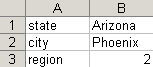Summary

This article describes how to use an Excel Lookup table to supply document parameter values.

Excel Requirements

Data Lookup

The Excel spreadsheet must contain a key column and a lookup column. The key column value is referenced to return the lookup value for that key. In the example below, state, city and region are key values.Date Lookup

The first column in the Excel spreadsheet must be labeled date, be formatted as DATE and contain a list of dates in format MM/DD/YYYY. The current date (burst execution date) is then used to look up a column containing date information. In the example below, start or end might be used as lookup values.Create a File List

Create a File List that references the Excel file containing the date lookup or data lookup information.
Assign the File List
• Select Burst Properties
• Select the Content Grouping and Lookups tab
• Select the ellipsis button next to the appropriate lookup type then select the File List

Reference the Lookup
• Select the parameter to receive values from the lookup
• Source Type: Select Enter Value
• Enter the lookup system macro:
• Data Lookup: [@LOOKUP(KEY)]
• Date Lookup: [@DATELOOKUP(COLUMN)]
• Select the Apply button